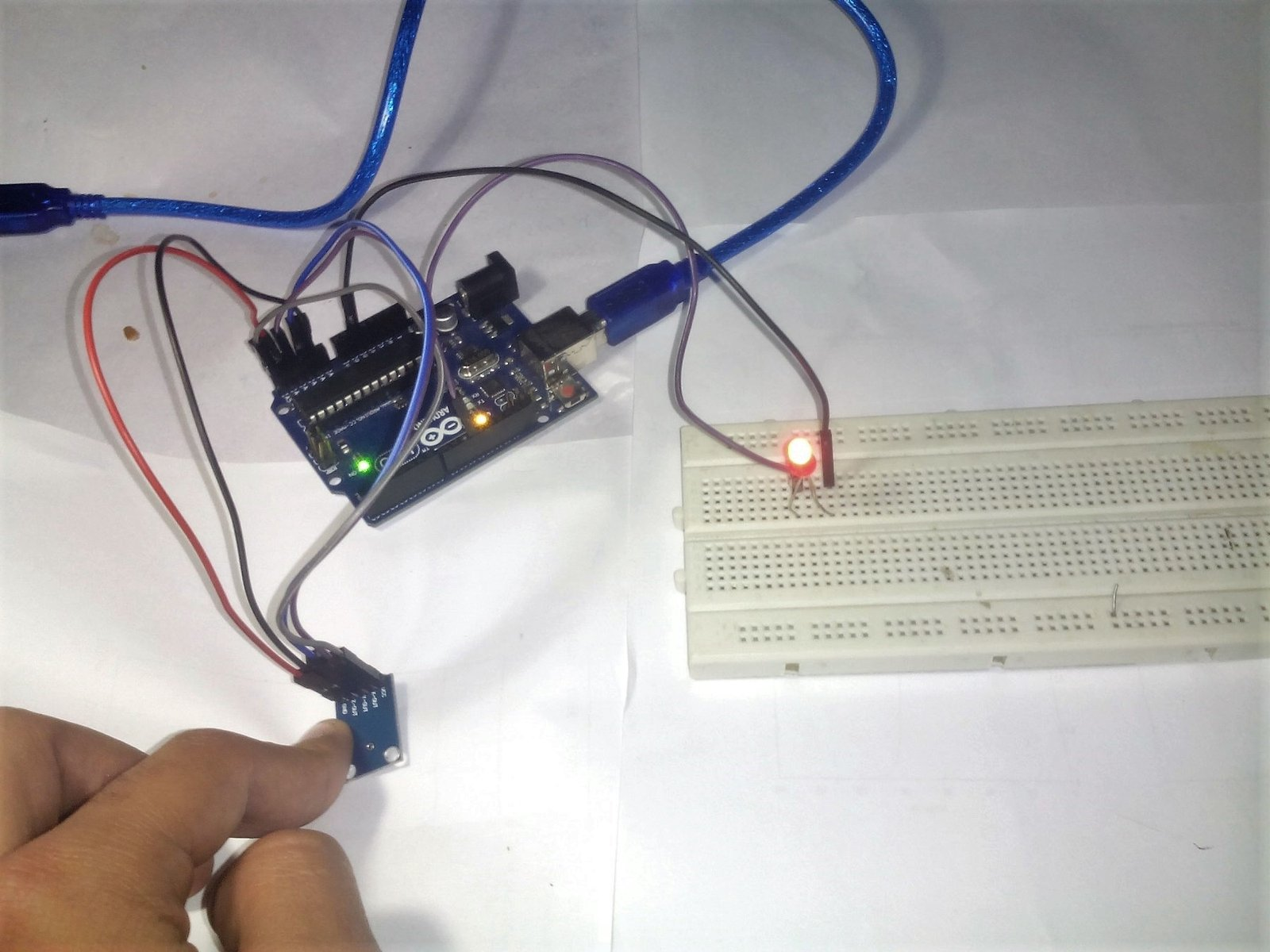# HOW TO INTERFACE AN ACCELEROMETER WITH ARDUINOIn this project we are going to control an led by Accelerometer sensor. Accelerometer is a sensor which calculates the three axis i.e. x,y, and z  axis in 3D space w.r.t a fixed origin. We can easily print value of each axis on Serial monitor of Arduino IDE.

LIST OF COMPONENTS REQUIRED

1. Arduino uno
2. Arduino software
4. Led
5. Accelerometer
6. Jumper wires

CIRCUIT DIAGRAM

CONNECTIONS

The circuit:

1. Gnd of the sensor to A4( analog input pin 4 of the arduino)
6. An led to pin 11 of arduino

CODE

UNDERSTANDING CODE:

In the void loop() function we are trying to take the readings of x, y, and z axis of the accelerometer and perform the desired task i.e. to switch the led  ON and OFF. We first replace the following code in void loop() of above code in order to take readings from the accelerometer. Than upload the code in arduino and turn serial monitor on of arduino software. Tilt the accelerometer in either direction the serial monitor will show the readings.

WORKING:

An accelerometer is a device that detects motion. It is available in two types

2 axis accelerometer and 3 axis accelerometer

Axis means the x, y and z direction in which the accelerometer is positioned.

This device gives the readings of x, y and z direcition on serial monitor option available in arduino software when positioned at different angles. We can thus note down the readings at different positions of accelerometer eg. tilted rightwards, tilted leftwards  and many other positions. Thus we can program it in a way that if accelerometer is tilted leftwards than led will be ON. Similarly we can control different devices using accelerometer.

### 5 Replies to “HOW TO INTERFACE AN ACCELEROMETER WITH ARDUINO”

1.Janel Treichel says:

Outstanding post however I was wanting to know if you could write a litte more on this subject? I’d be very grateful if you could elaborate a little bit more. Thanks!

1.Ankit Negi says:

2.Maryann Say says:
3.Garfield says:
4.minecraft says: